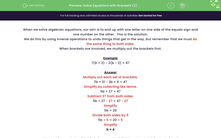# Solve Equations with Brackets (2)

In this worksheet, students solve equations with two sets of brackets.Key stage:  KS 3

Curriculum topic:   Algebra

Curriculum subtopic:   Solve Linear Equations (One Variable)

Difficulty level:#### Worksheet Overview

When we solve algebraic equations, our aim is to end up with one letter on one side of the equals sign and one number on the other.  This is the solution.

We do this by using inverse operations to undo things that get in the way, but remember that we must do the same thing to both sides.

When brackets are involved, we multiply out the brackets first.

Example

7(b + 3) - 2(b - 3) = 47

Multiply out each set of brackets.

7b + 21 - 2b + 6 = 47

Simplify by collecting like terms.

5b + 27 = 47

Subtract 27 from both sides.

5b + 27 - 27 = 47 - 27

Simplify

5b = 20

Divide both sides by 5

5b ÷ 5 = 20 ÷ 5

Simplify

b = 4

Want a bit more help with this before you begin? Why not watch this short video?

### What is EdPlace?

We're your National Curriculum aligned online education content provider helping each child succeed in English, maths and science from year 1 to GCSE. With an EdPlace account you’ll be able to track and measure progress, helping each child achieve their best. We build confidence and attainment by personalising each child’s learning at a level that suits them.

Get started